nextnano.com  Tool: nextnano++  Tool: nextnano³  Download | Search | Copyright | Publications  * password protected nextnano³ software1D bulk k.p dispersion in GaN

# nextnano3 - Tutorial

## k.p dispersion in bulk unstrained, compressively and tensilely strained GaN (wurtzite)

Author: Stefan Birner

If you want to obtain the input files that are used within this tutorial, please check if you can find them in the installation directory.
If you cannot find them, please submit a Support Ticket.
```-> bulk_kp_dispersion_GaN_unstrained_0_nn3.in           / *_nnp.in - ```input file for nextnano3 / nextnano++ software``` -> bulk_kp_dispersion_GaN_unstrained_90_nn3.in          / *_nnp.in - ```input file for nextnano3 / nextnano++ software``` -> bulk_kp_dispersion_GaN_strain_compressive_0_nn3.in   / *_nnp.in - ```input file for nextnano3 / nextnano++ software``` -> bulk_kp_dispersion_GaN_strain_compressive_90_nn3.in  / *_nnp.in - ```input file for nextnano3 / nextnano++ software``` -> bulk_kp_dispersion_GaN_strained_tensile_0_nn3.in     / *_nnp.in - ```input file for nextnano3 / nextnano++ software``` -> bulk_kp_dispersion_GaN_strained_tensile_90_nn3.in    / *_nnp.in - ```input file for nextnano3 / nextnano++ software``` -> bulk_kp_dispersion_GaN_strained_tensile_90_3D_nn3.in / *_nnp.in - ```input file for nextnano3 / nextnano++ software```  ```

This tutorial is based on

`[ParkChuangPRB1999]`
Crystal-orientation effects on the piezoelectric field and electronic properties of strained wurtzite semiconductors

S.-H. Park, S.-L. Chuang
Phys. Rev. B 59, 4725 (1999).

Further material parameters used in the calculations are taken from

`[KumagaiChuangAndoPRB1998]`
Analytical solutions of the block-diagonalized Hamiltonian for strained wurtzite semiconductors
M. Kumagai, S. L. Chuang, H. Ando
Phys. Rev. B 57, 15303 (1998).

We calculate E(k) for bulk GaN (unstrained), with compressive and with tensile strain, along two different growth directions.

## k.p dispersion in bulk unstrained GaN (wurtzite)

• We want to calculate the dispersion E(k) from |k|=0 to |k|=1.0 [1/nm] along the following directions in k space:
-  to 
-  to 
-  to 
We compare 6-band k.p theory results vs. single-band (effective-mass) results for unstrained GaN.

### Calculating the bulk k.p dispersion

• ``` \$output-kp-data  destination-directory  = kp/  bulk-kp-dispersion     = yes              ! ```calculate bulk k.p dispersion```  grid-position          = 2.0              ! ```in units of` [nm]````  !----------------------------------------  ! Dispersion along  direction  ! maximum |k| vector = 1.2 [1/nm]  !----------------------------------------  k-direction-to-k-point = 1.2   0.0   0.0  ! ```k direction and range for dispersion plot``` [1/nm]                                            ! ```related to output file``` kp_bulk/bulk_6x6kp_dispersion_as_in_inputfile_kxkykz_000_kxkykz.dat  number-of-k-points     = 100 ! ```number of k points to be calculated (resolution)
The maximum value of |k| is 1.2 [1/nm].
Note that for values of |k| larger than 1.0 [1/nm], k.p theory might not be a good approximation any more.
• We calculate the pure bulk dispersion at` grid-position = 2.0`, i.e. for the material located at the grid point at 2 nm.
In our case this is GaN but it could be any strained alloy. If strain is present (see below), the k.p Bir-Pikus strain Hamiltonian will be diagonalized at each k point.
The grid point at` grid-position `must be located inside a quantum cluster.
`shift-holes-to-zero = yes `forces the top of the valence band to be located at 0 eV.
In this tutorial, however, we use` no`.
Out zero point of energy is the "average" energy of all three hole bands.
Here, "average" means without taking crystal field and spin-orbit splitting into account. This is added afterwards to get the energies of heavy hole (HH), light hole (LH) and crystal-field split-off hole (CH).
How often the bulk k.p Hamiltonian should be solved can be specified via` number-of-k-points`. To increase the resolution, just increase this number.
• The results can be found in:```  kp_bulk/bulk_6x6kp_dispersion_as_in_inputfile_kxkykz_000_kxkykz.dat ``` (6-band k.p)  `- `along the path from  to  (Gamma point) and to ```  kp_bulk/bulk_sg_dispersion.dat                         ```(single-band approximation)``` - ```along the path from  to  (Gamma point) and to 
` kp/bulk_sg_dispersion.dat                              `(single-band approximation)``` - ```along the path from  to  (Gamma point) and to 

The following figure shows the bulk k.p dispersion of unstrained GaN (wurtzite).
The kz direction corresponds to the c axis .
The dispersion along kx and ky is identical (only kx is shown), i.e. the dispersion in the (001) plane is isotropic.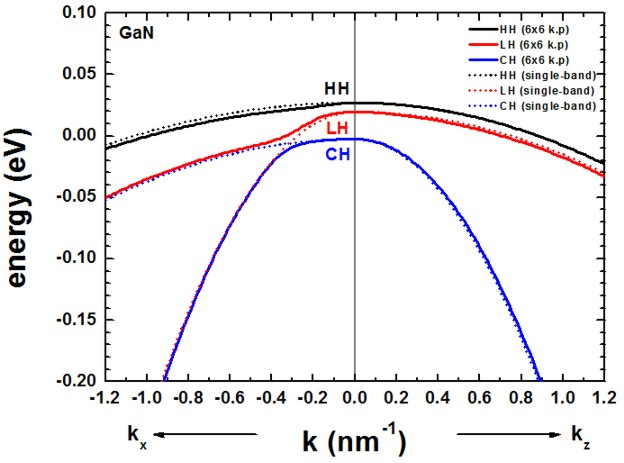The results of our figure is in excellent agreement to Fig. 4 (b) of the paper `[KumagaiChuangAndoPRB1998]`.

• The dispersion along the hexagonal c axis is substantially different.

If the average of the three valence band edges (without taking crystal-field and spin-orbit splitting into account) is defined to be at zero, i.e. Ev,av = 0 eV, then the energies E1, E2 and E3 are defined as follows for the unstrained case:

E1 = Delta1 + Delta2
E2 = B + A
E3 = B `-` A

where Delta1 is the crystal field split energy Deltacr, and Delta2 and Delta3 are related to the spin-orbit split off-energy Deltaso as follows:
Delta2 = Delta3 = 1/3 Deltaso
B = (Delta1 `-` Delta2)/ 2.
A = SQRT(B2 + 2 Delta32)

The Delta parameters are defined in the database
```  6x6-kp-parameters = ... 0.0220 0.005 0.005 ! Delta1(cr),Delta2=Delta_so/3,Delta3=Delta_so/3 ```leading to:

B = 0.0085
A = 0.01106
E1 = Delta1 + Delta2                 =   0.027     eV
E2 = B + A =   0.0085 + 0.01106 =   0.01956 eV
E3 = B `-` A =   0.0085 `-` 0.01106 = `-`0.00256 eV

• In contrast to zinc blende materials, even in the unstrained case, the heavy and light hole are not degenerate at k = 0.
• For comparison, we also show the dispersion using the single-band effective mass approximation (dotted lines).
We used the following values for the effective hole masses, according to reference http://www.ioffe.rssi.ru/SVA/NSM/Semicond/GaN/bandstr.html.
mHH,a = 1.6`  [m0]`, mHH,c = 1.1``` [m0] ```mLH,a = 0.15` [m0]`, mLH,c = 1.1``` [m0] ```mCH,a = 1.1 ` [m0]`, mCH,c = 0.15``` [m0] `````` valence-band-masses = 1.6  1.6  1.1  ! HH m_|_, m_|_, m|| (with respect to c-axis)                        0.15 0.15 1.1  ! LH m_|_, m_|_, m|| (with respect to c-axis)                        1.1  1.1  0.10 ! CH m_|_, m_|_, m|| (with respect to c-axis)```
• The effective mass approximation is a simple parabolic dispersion which is isotropic in zinc blende materials (i.e. no dependence on the k vector direction) but is anisotropic for wurtzite materials due to the different effective masses paralllel and perpendicular to the c axis.

## k.p dispersion in compressively and tensilely strained GaN (wurtzite)

• We compare two different orientations of the crystal coordiate system with respect to the simulation coordinate system.
Case a) Default orientation: hexagonal c axis oriented along the z direction 
Case b) Rotation of hexagonal c axis by 90 degrees so that it oriented along the default x direction [10-10]
The orientation of the y axis remains the same.

``` \$domain-coordinates   ...   growth-coordinate-axis = 0 0 1 ! ``` along z direction of simulation coordinate system``` ```Case a)```  !-----------------------------------------------------------------------------------------  ! The angle theta is defined as the angle between the growth direction z' and the c axis.  ! Angle theta = 0° corresponds to the z' =  growth direction.  !-----------------------------------------------------------------------------------------   hkil-x-direction =  1 0 -1 0 ! Miller Indices of x-coordinate axes direction (DEFAULT)  !hkil-y-direction = -1 2 -1 0 ! Miller Indices of y-coordinate axes direction (DEFAULT)   hkil-z-direction =  0 0  0 1 ! Miller Indices of z-coordinate axes direction (DEFAULT)  !----------------------------------------------------------------------------------------- ```Case b)```  !-----------------------------------------------------------------------------------------  ! Angle theta = 90° corresponds to the z' = [10-10] growth direction.  !-----------------------------------------------------------------------------------------   hkil-x-direction =  0 0  0 -1 ! Miller Indices of x-coordinate axes direction  !hkil-y-direction = -1 2 -1  0 ! Miller Indices of y-coordinate axes direction   hkil-z-direction =  1 0 -1  0 ! Miller Indices of z-coordinate axes direction !-----------------------------------------------------------------------------------------```

The following figures compare the 6-band k.p valence band dispersion relation of compressively (`-`0.5%, figure on the left) vs. tensilely (+0.5%, figure on the right) strained GaN.
Assuming that the substrate material is Al(x)In(1-x)N,
`- `a compressive strain of `-`0.5% corresponds to Al0.785In0.215N (exx = eyy = `-`0.005)
`- `a tensile strain of 0.5% corresponds to Al0.859In0.141N (exx = eyy = 0.005)
using the lattice constants given in the references cited above.
The results for tensile strain indicate that the light hole (LH) band is higher in energy than the heavy hole (HH) band.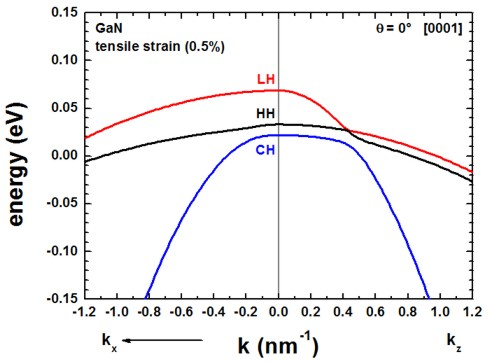The results of these two figures can be found in this file:``` kp/bulk_6x6kp_dispersion_100_000_001.dat ```where` 100 `represents the kx direction,``` 000 ```the Gamma point and` 001 `the kz direction, i.e. the plotted dispersion is a cut through the 3D Brillouin zone along these lines.
We only plotted the result for kx. The dispersion along ky is identical in this case. Also the dispersion along , i.e. the dispersion is isotropic with respect to the (001) plane.

Once the c axis is oriented along the z axis of the simulation coordinate system (rotation by 90 degrees around the y axis, c axis along [10-10] direction),
the corresponding results look as follows.
Actually the` .log `file (screen output) of the simulation indicates the rotation angle and the rotation axis:
```  ==> rotation angle =  90.000000000 ° = 0.500000000 [pi] = 1.570796327   ==> rotation axis  = [ 0.000000000 1.000000000 0.000000000 ]```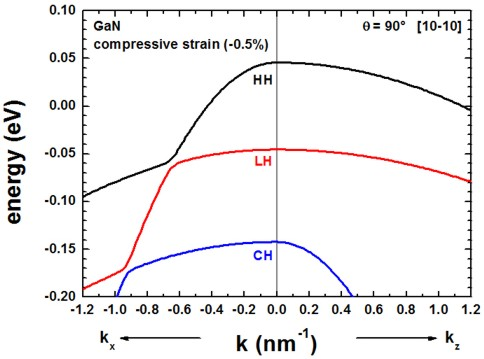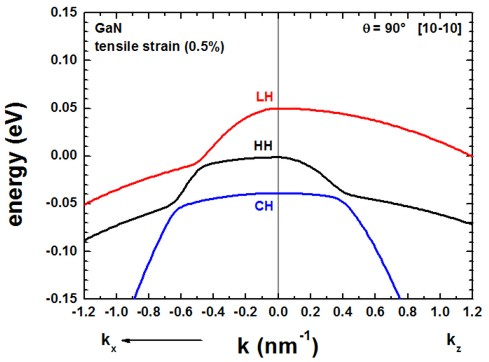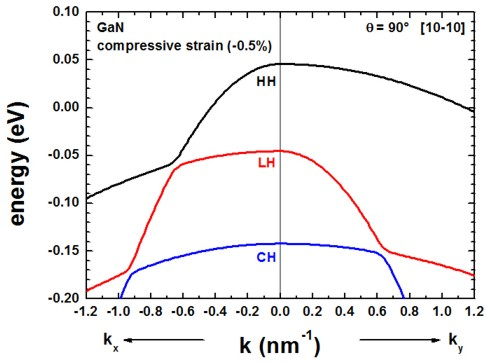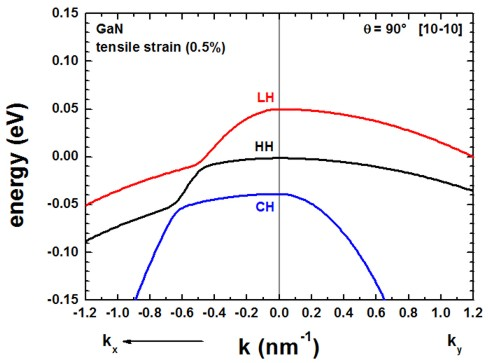The results of these two figures can be found in this file:``` kp/bulk_6x6kp_dispersion_100_000_001.dat ```where` 100 `represents the kx direction,``` 000 ```the Gamma point and` 001 `the kz direction, i.e. the plotted dispersion is a cut through the 3D Brillouin zone along these lines.
The results for the dispersion along ky is now different from the dispersion along kx.
The results for ky are contained in this file
```kp/bulk_6x6kp_dispersion_000_kxkykz.dat ```because here we specified in the input file to calculate the dispersion from the Gamma point (0,0,0) to (kx,ky,kz) = (0 , 1.2 nm-1 , 0).
```  \$output-kp-data    k-direction-to-k-point = 0.0 1.2 0.0 ! ``` (kx,ky,kz) in units of` [1/nm]`

Note: For theta = 90°, we have rotated the crystal (cr) coordinate system with respect to the simulation (sim) coordinate system.
Therfore, for our new orientation it holds eyy,cr = eyy,sim = +`-`0.005 and exx /= eyy.

The results of our figures are in excellent agreement to figures 5 and 6 of the paper `[ParkChuangPRB1999]`.

Note that for the case of tensile strain and orientation of the c axis along the [10-10] orientation, the strain tensor component along the y direction of the simulation system is tensilely strained whereas the component along the x direction is compressively (!) strained.

For a discussion of the figures please refer to the paper of Park and Chuang.

### Energy dispersion E(k) in three dimensions

Alternatively one can print out the 3D data field of the bulk E(k) = E(kx,ky,kz) dispersion.

``` \$output-kp-data  ...  bulk-kp-dispersion-3D  = yes  grid-position          = 2.0              ! ```in units of``` [nm]!---------------------------------------- ! maximum |k| vector = 1.2 [1/nm] !----------------------------------------  k-direction-to-k-point = 1.2 0.0 0.0      ! ```k-direction and range for dispersion plot``` [1/nm]  number-of-k-points     = 20               ! ```number of k points to calculated (resolution)

The meaning of``` number-of-k-points = 20 ```is the following:
20 k points from '```- maximum |k| vector' ```to zero (plus the Gamma point) and
20 k points from zero to  '`+ maximum |k| vector'` (plus the Gamma point) along all three directions,
i.e. the whole 3D volume then contains 21 * 21 * 21 = 9261 k points.

Left: A 2D slice in the (kx,ky) plane for kz=0 of the highest lying hole state for the tensilely strained GaN (oriented along 90 degrees, i.e. z is oriented along [10-10]) is shown in this figure.
Right: Horizontal and vertical slice through the center coordinate at (kx,ky,kz) = (0,0,0).If you need support, or input files that are not included in the installation folder, or if you have suggestions on how we can improve our website or tutorials, please submit a Support Ticket.